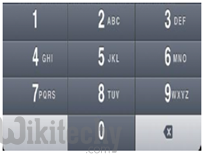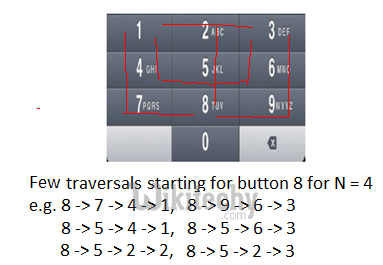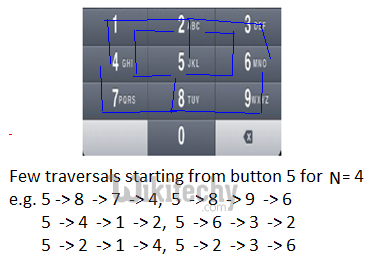Mobile Numeric Keypad Problem - Dynamic Programming Given the mobile numeric keypad. You can only press buttons that are up, left, right or downGiven the mobile numeric keypad. You can only press buttons that are up, left, right or down to the current button. You are not allowed to press bottom row corner buttons (i.e. * and # ).
Given a number N, find out the number of possible numbers of given length.

Examples:
For N=1, number of possible numbers would be 10 (0, 1, 2, 3, …., 9)
For N=2, number of possible numbers would be 36
Possible numbers: 00,08 11,12,14 22,21,23,25 and so on.
If we start with 0, valid numbers will be 00, 08 (count: 2)
If we start with 1, valid numbers will be 11, 12, 14 (count: 3)
If we start with 2, valid numbers will be 22, 21, 23,25 (count: 4)
If we start with 3, valid numbers will be 33, 32, 36 (count: 3)
If we start with 4, valid numbers will be 44,41,45,47 (count: 4)
If we start with 5, valid numbers will be 55,54,52,56,58 (count: 5)
………………………………
………………………………

We need to print the count of possible numbers.

We strongly recommend to minimize the browser and try this yourself first.

N = 1 is trivial case, number of possible numbers would be 10 (0, 1, 2, 3, …., 9)
For N > 1, we need to start from some button, then move to any of the four direction (up, left, right or down) which takes to a valid button (should not go to *, #). Keep doing this until N length number is obtained (depth first traversal).

Recursive Solution:
Mobile Keypad is a rectangular grid of 4X3 (4 rows and 3 columns)
Lets say Count(i, j, N) represents the count of N length numbers starting from position (i, j)

```If N = 1
Count(i, j, N) = 10
Else
Count(i, j, N) = Sum of all Count(r, c, N-1) where (r, c) is new
position after valid move of length 1 from current
position (i, j)```

Following is C implementation of above recursive formula.

C
``````// A Naive Recursive C program to count number of possible numbers
// of given length
#include <stdio.h>

// left, up, right, down move from current location
int row[] = {0, 0, -1, 0, 1};
int col[] = {0, -1, 0, 1, 0};

// Returns count of numbers of length n starting from key position
// (i, j) in a numeric keyboard.
int getCountUtil(char keypad[], int i, int j, int n)
{
if (keypad == NULL || n <= 0)
return 0;

// From a given key, only one number is possible of length 1
if (n == 1)
return 1;

int k=0, move=0, ro=0, co=0, totalCount = 0;

// move left, up, right, down from current location and if
// new location is valid, then get number count of length
// (n-1) from that new position and add in count obtained so far
for (move=0; move<5; move++)
{
ro = i + row[move];
co = j + col[move];
if (ro >= 0 && ro <= 3 && co >=0 && co <= 2 &&
{
totalCount += getCountUtil(keypad, ro, co, n-1);
}
}

}

// Return count of all possible numbers of length n
// in a given numeric keyboard
{
// Base cases
if (keypad == NULL || n <= 0)
return 0;
if (n == 1)
return 10;

int i=0, j=0, totalCount = 0;
for (i=0; i<4; i++)  // Loop on keypad row
{
for (j=0; j<3; j++)   // Loop on keypad column
{
// Process for 0 to 9 digits
{
// Get count when number is starting from key
// position (i, j) and add in count obtained so far
totalCount += getCountUtil(keypad, i, j, n);
}
}
}
}

// Driver program to test above function
int main(int argc, char *argv[])
{
{'4','5','6'},
{'7','8','9'},
{'*','0','#'}};
printf("Count for numbers of length %d: %d\n", 1, getCount(keypad, 1));
printf("Count for numbers of length %d: %d\n", 2, getCount(keypad, 2));
printf("Count for numbers of length %d: %d\n", 3, getCount(keypad, 3));
printf("Count for numbers of length %d: %d\n", 4, getCount(keypad, 4));
printf("Count for numbers of length %d: %d\n", 5, getCount(keypad, 5));

return 0;
}``````

Output:

```Count for numbers of length 1: 10
Count for numbers of length 2: 36
Count for numbers of length 3: 138
Count for numbers of length 4: 532
Count for numbers of length 5: 2062
```

Dynamic Programming
There are many repeated traversal on smaller paths (traversal for smaller N) to find all possible longer paths (traversal for bigger N). See following two diagrams for example. In this traversal, for N = 4 from two starting positions (buttons ‘4’ and ‘8’), we can see there are few repeated traversals for N = 2 (e.g. 4 -> 1, 6 -> 3, 8 -> 9, 8 -> 7 etc).Since the problem has both properties: Optimal Substructure and Overlapping Subproblems, it can be efficiently solved using dynamic programming.

Following is C program for dynamic programming implementation.

C
``````// A Dynamic Programming based C program to count number of
// possible numbers of given length
#include <stdio.h>

// Return count of all possible numbers of length n
// in a given numeric keyboard
{
if(keypad == NULL || n <= 0)
return 0;
if(n == 1)
return 10;

// left, up, right, down move from current location
int row[] = {0, 0, -1, 0, 1};
int col[] = {0, -1, 0, 1, 0};

// taking n+1 for simplicity - count[i][j] will store
// number count starting with digit i and length j
int count[n+1];
int i=0, j=0, k=0, move=0, ro=0, co=0, num = 0;
int nextNum=0, totalCount = 0;

// count numbers starting with digit i and of lengths 0 and 1
for (i=0; i<=9; i++)
{
count[i] = 0;
count[i] = 1;
}

// Bottom up - Get number count of length 2, 3, 4, ... , n
for (k=2; k<=n; k++)
{
for (i=0; i<4; i++)  // Loop on keypad row
{
for (j=0; j<3; j++)   // Loop on keypad column
{
// Process for 0 to 9 digits
{
// Here we are counting the numbers starting with
// will become 1st digit, and we need to look for
// (k-1) more digits
count[num][k] = 0;

// move left, up, right, down from current location
// and if new location is valid, then get number
// count of length (k-1) from that new digit and
// add in count we found so far
for (move=0; move<5; move++)
{
ro = i + row[move];
co = j + col[move];
if (ro >= 0 && ro <= 3 && co >=0 && co <= 2 &&
{
count[num][k] += count[nextNum][k-1];
}
}
}
}
}
}

// Get count of all possible numbers of length "n" starting
// with digit 0, 1, 2, ..., 9
totalCount = 0;
for (i=0; i<=9; i++)
totalCount += count[i][n];
}

// Driver program to test above function
int main(int argc, char *argv[])
{
{'4','5','6'},
{'7','8','9'},
{'*','0','#'}};
printf("Count for numbers of length %d: %d\n", 1, getCount(keypad, 1));
printf("Count for numbers of length %d: %d\n", 2, getCount(keypad, 2));
printf("Count for numbers of length %d: %d\n", 3, getCount(keypad, 3));
printf("Count for numbers of length %d: %d\n", 4, getCount(keypad, 4));
printf("Count for numbers of length %d: %d\n", 5, getCount(keypad, 5));

return 0;
}``````

Output:

```Count for numbers of length 1: 10
Count for numbers of length 2: 36
Count for numbers of length 3: 138
Count for numbers of length 4: 532
Count for numbers of length 5: 2062```

A Space Optimized Solution:
The above dynamic programming approach also runs in O(n) time and requires O(n) auxiliary space, as only one for loop runs n times, other for loops runs for constant time. We can see that nth iteration needs data from (n-1)th iteration only, so we need not keep the data from older iterations. We can have a space efficient dynamic programming approach with just two arrays of size 10. Thanks to Nik for suggesting this solution.

C
``````// A Space Optimized C program to count number of possible numbers
// of given length
#include <stdio.h>

// Return count of all possible numbers of length n
// in a given numeric keyboard
{
if(keypad == NULL || n <= 0)
return 0;
if(n == 1)
return 10;

// odd[i], even[i] arrays represent count of numbers starting
// with digit i for any length j
int odd, even;
int i = 0, j = 0, useOdd = 0, totalCount = 0;

for (i=0; i<=9; i++)
odd[i] = 1;  // for j = 1

for (j=2; j<=n; j++) // Bottom Up calculation from j = 2 to n
{
useOdd = 1 - useOdd;

// Here we are explicitly writing lines for each number 0
// to 9. But it can always be written as DFS on 4X3 grid
// using row, column array valid moves
if(useOdd == 1)
{
even = odd + odd;
even = odd + odd + odd;
even = odd + odd + odd + odd;
even = odd + odd + odd;
even = odd + odd + odd + odd;
even = odd + odd + odd + odd + odd;
even = odd + odd + odd + odd;
even = odd + odd + odd;
even = odd + odd + odd + odd + odd;
even = odd + odd + odd;
}
else
{
odd = even + even;
odd = even + even + even;
odd = even + even + even + even;
odd = even + even + even;
odd = even + even + even + even;
odd = even + even + even + even + even;
odd = even + even + even + even;
odd = even + even + even;
odd = even + even + even + even + even;
odd = even + even + even;
}
}

// Get count of all possible numbers of length "n" starting
// with digit 0, 1, 2, ..., 9
totalCount = 0;
if(useOdd == 1)
{
for (i=0; i<=9; i++)
totalCount += even[i];
}
else
{
for (i=0; i<=9; i++)
totalCount += odd[i];
}
}

// Driver program to test above function
int main()
{
{'4','5','6'},
{'7','8','9'},
{'*','0','#'}
};
printf("Count for numbers of length %d: %d\n", 1, getCount(keypad, 1));
printf("Count for numbers of length %d: %d\n", 2, getCount(keypad, 2));
printf("Count for numbers of length %d: %d\n", 3, getCount(keypad, 3));
printf("Count for numbers of length %d: %d\n", 4, getCount(keypad, 4));
printf("Count for numbers of length %d: %d\n", 5, getCount(keypad, 5));

return 0;
}``````

Output:

```Count for numbers of length 1: 10
Count for numbers of length 2: 36
Count for numbers of length 3: 138
Count for numbers of length 4: 532
Count for numbers of length 5: 2062```